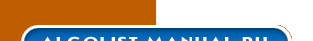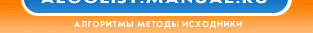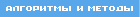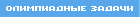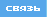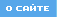╧ґҐ№: ╠рҐхьрҐшър » ├хюьхҐЁш  » ┬√яґъыр  юсюыюіър » QuickHull 3D
QuickHull 3DJordan Smith

The Quick Hull is a fairly easy to understand algorithm for finding the convex hull in d dimensions. The following is a description of how it works in 3 dimensions. I have added extra information on how to find the horizon contour of a polyhedron as seen from a point which is known to be outside of a particular face of the polyhedron [Seidel94]. I have also added information on how to merge coplanar faces of the polyhedron, which is necessary to have clean face binding relationships as defined by the CDA structure. And I also do coincident vertex merging of vertices on the convex hull.

The input to the algorithm is a list of vertices where each vertex points to at most one primal face which it is the dual of. The output is the convex hull which is a polyhedron whose vertices are linked to the primal faces which created them. In some cases a single vertex will point to two primal faces.

``` Polyhedron QUICK_HULL(List of Vertices listVertices) Allocate a Polyhedron which will be our return value. Find a maximal simplex of the current dimension. In our case, find 4 points which define maximal tetrahedron, and create the tetrahedron which will be our seed partial answer. CREATE_SIMPLEX(Polyhedron, listVertices). If this returns a dimension lower than 3, report the degeneracy and quit. Divide the points of the cloud into the outside sets of the four faces of the tetrahedron. This is done by calling ADD_TO_OUTSIDE_SET(face, listVertices) on each of the 4 faces in turn. Points that could be put in multiple sets will be randomly placed in one of them, and it does not matter which one. Any points which are inside or on all of the planes do not contribute to the convex hull. These points are removed from the rest of the calculation. While ( There exists currFace = a face on the current convex hull which has a non-empty outside set of vertices ) Find the point in currFace's outside set which is farthest away from the plane of the currFace. This is the eyePoint. Compute the horizon of the current polyhedron as seen from this eyePoint. CALCULATE_HORIZON(eyePoint, NULL, currFace, listHorizonEdges, listUnclaimedVertices). This will mark all of the visible faces as not on the convex hull and place all of their outside set points on the listUnclaimedVertices. It is creates the listHorizonEdges which is a counterclockwise ordered list of edges around the horizon contour of the polyhedron as viewed from the eyePoint. Construct a cone from the eye point to all of the edges of the horizon. Before a triangle of this cone is created and added to the polyhedron a test to see if it coplanar to the face on the other side of the horizon edge is applied. If the faces are coplanar then they are merged together and that horizon edge is marked as not on the convex hull. Then a similar check must also be applied to edges which cross the horizon to see if they are colinear. If so then the vertex on the horizon which is on this line must be marked as not on the horizon. Divide the points of the listUnclaimedVertices into the outside sets of the new cone faces and the faces which were merged with what would be new cone faces. This is done by calling ADD_TO_OUTSIDE_SET(face, listUnclaimedVertices) on each of these faces in turn. Points that could be put in multiple sets will be randomly placed in one of them, and it does not matter which one. Any points which are inside or on all of the planes do not contribute to the convex hull. These points are marked as not on the convex hull. Remove all faces, edges, and vertices which have been marked as not on the convex hull. These faces, edges, and vertices have all been enclosed by the new cone emanating from the eyePoint. Continue the loop When all the faces with outside points have been processed we are done. The polyhedron should a clean convex hull where all coplanar faces have been merged. All colinear edges have been merged. And all coincident vertices have had their DualFace pointers merged. ```

### Creating an Initial Simplex

The CREATE_SIMPLEX helper function finds a hopefully maximal tetrahedron to start the algorithm with. The function returns a polyhedron which is the simplex and a value which is the dimension of the simplex. If the dimension < 3 than we have a degenerate point cloud, and we should recurse on dimension to find the convex hull in the lower dimension.

``` Dimension CREATE_SIMPLEX(Polyhedron, listVertices) For each principle direction (X-axis, Y-axis, Z-axis) Find the minimum and maximum point in that dimension If min != max then break out of loop with success Continue the loop If the loop finished without success return and report that we have a degenerate point cloud, either 1D or 2D. Find the point which is furthest distant from the line defined by the first two points. If this maximum distance is zero, then our point cloud is 1D, so report the degeneracy. Find the point which has the largest absolute distance from the plane defined by the first three points. If this maximum distance is zero, then our point cloud is 2D, so report the the degeneracy. Create the tetrahedron polyhedron. Remember that if the distance from the plane of the fourth point was negative, then the order of the first three vertices must be reversed. Return the fact that we created a non-degenerate tetrahedron of dimension 3. ```

### Adding Points to a Face's Outside Set

The ADD_TO_OUTSIDE_SET helper function is reused many times, and it is also the place where I detect and handle coincident vertex merging. This merging is necessary for tracking face bindings through the duality mapping that happens in the INTERSECT algorithm.

``` Void ADD_TO_OUTSIDE_SET(Face face, List of unclaimed Vertices listVertices) For each vertex in the listVertices distance = Distance from the plane of the face to the vertex If the vertex is in the plane of the face (i.e. distance == 0) Then check to see if the vertex is coincident with any vertices of the face, and if so merge it into that face vertex. Else If distance > 0 Then claim the vertex by removing it from this list and adding it the face's set of outside vertices Continue the loop ```

### Calculating the Horizon Contour

The CALCULATE_HORIZON helper function was not well described by the Quick Hull paper. I actually learned the trick for computing the horizon edges from some old notes from one of Seidel's classes which Sara McMains showed to me. The trick is the Depth First Search described in the algorithm which not only finds the horizon edges, but also reports them in counterclockwise order. We start at the face for which the eyePoint was a member of the outside set.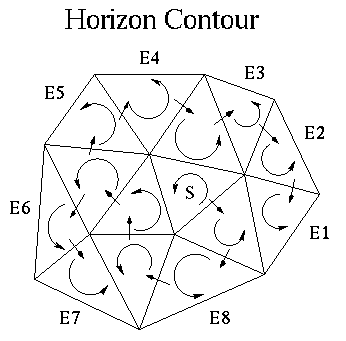``` Void CALCULATE_HORIZON(eyePoint, crossedEdge, currFace, listHorizonEdges, listUnclaimedVertices) If the currFace is not on the convex hull Mark the crossedEdge as not on the convex hull and return. ```
• ```If the currFace is visible from the eyePoint Mark the currFace as not on the convex hull. Remove all vertices from the currFace's outside set, and add them to the listUnclaimedVertices. c. If the crossedEdge != NULL (only for the first face) then mark the crossedEdge as not on the convex hull d. Cross each of the edges of currFace which are still on the convex hull in counterclockwise order starting from the edge after the crossedEdge (in the case of the first face, pick any edge to start with). For each currEdge recurse with the call. ```

•   References• [Barber93] Barber, C. B., Dobkin, D. P., Huhdanpaa, H. T., The Quickhull algorithm for convex hull, GCG53, The Geometry Center, Minneapolis, 1993 (ftp.geom.umn.edu/pub/software/qhull.tar.Z)
• [de Berg97] de Berg, M., van Krevald, M., Overmars, M., Schwarzkopf, O., Computational Geometry: Algorithms and Applications, Springer-Verlag, Berlin, Heidelberg, 1997
• [Preparata85] Preparata, F., Shamos, M. I., Computational Geometry: An Introduction, Springer-Verlag, New York, 1985
• [Seidel91] Small-dimensional linear programming and convex hulls made easy, Discrete Computational Geometry, 6:423-434, 1991